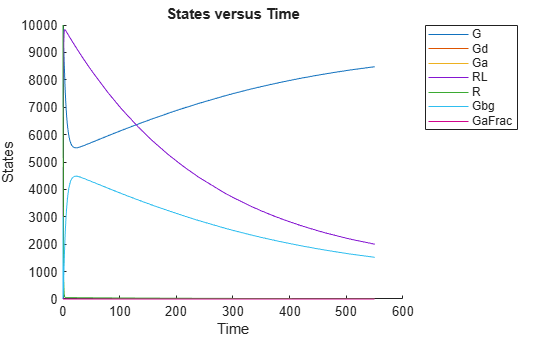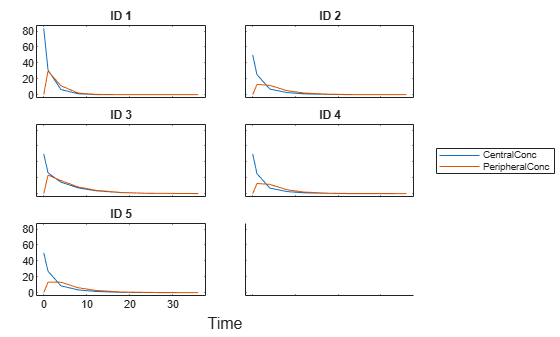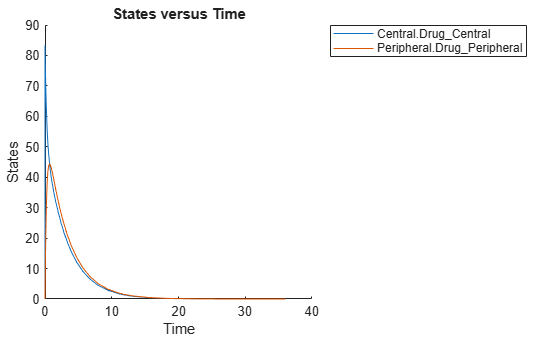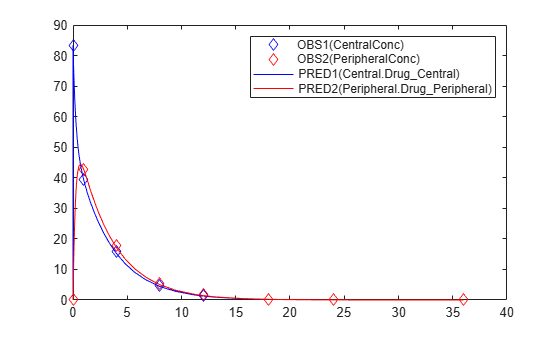# predict

Simulate and evaluate fitted SimBiology model

## Syntax

``````[ynew,parameterEstimates]= predict(resultsObj)``````
``````[ynew,parameterEstimates]= predict(resultsObj,data,dosing)``````
``````[ynew,parameterEstimates]= predict(resultsObj,data,dosing,'Variants',v)``````

## Description

example

``````[ynew,parameterEstimates]= predict(resultsObj)``` returns simulation results `ynew` and parameter estimates `parameterEstimates` of a fitted SimBiology® model.```

example

``````[ynew,parameterEstimates]= predict(resultsObj,data,dosing)``` returns simulation results `ynew` and estimated parameter values `parameterEstimates` from evaluating the fitted SimBiology model using the specified `data` and `dosing` information.During simulations, `predict` uses the parameter values in the `resultsObj.ParameterEstimates` property. Use this method when you want to evaluate the fitted model and predict responses using new data and/or dosing information.```
``````[ynew,parameterEstimates]= predict(resultsObj,data,dosing,'Variants',v)``` simulates the fitted model and applies the specified variants to each simulation.```

## Examples

collapse all

This example uses the yeast heterotrimeric G protein model and experimental data reported by . For details about the model, see the Background section in Parameter Scanning, Parameter Estimation, and Sensitivity Analysis in the Yeast Heterotrimeric G Protein Cycle.

`sbioloadproject gprotein`

Store the experimental data containing the time course for the fraction of active G protein.

```time = [0 10 30 60 110 210 300 450 600]'; GaFracExpt = [0 0.35 0.4 0.36 0.39 0.33 0.24 0.17 0.2]';```

Map the appropriate model component to the experimental data. In other words, indicate which species in the model corresponds to which response variable in the data. In this example, map the model parameter `GaFrac` to the experimental data variable `GaFracExpt` from `grpData`.

`responseMap = 'GaFrac = GaFracExpt';`

Create a `groupedData` object based on the experimental data.

```tbl = table(time,GaFracExpt); grpData = groupedData(tbl);```

Use an `estimatedInfo` object to define the model parameter `kGd` as a parameter to be estimated.

`estimatedParam = estimatedInfo('kGd');`

Perform the parameter estimation.

`fitResult = sbiofit(m1,grpData,responseMap,estimatedParam);`

View the estimated parameter value of `kGd`.

`fitResult.ParameterEstimates`
```ans=1×3 table Name Estimate StandardError _______ ________ _____________ {'kGd'} 0.11307 3.4439e-05 ```

Suppose you want to simulate the fitted model using different output times than those in the training data. You can use the `predict` method to do so.

Create a new variable `T` with different output times.

`T = [0;10;50;80;100;150;300;350;400;450;500;550];`

Use the `predict` method to simulate the fitted model on the new time points. No dosing was specified when you first ran `sbiofit`. Hence, you cannot use any dosing information with the `predict` method, and an empty array must be specified as the third input argument.

`ynew = predict(fitResult,T,[]);`

Plot the simulated data with the new output times.

`sbioplot(ynew);`This example shows how to estimate category-specific (such as young versus old, male versus female) PK parameters using the profile data from multiple individuals using a two-compartment model. The parameters to estimate are the volumes of central and peripheral compartment, the clearance, and intercompartmental clearance.

The synthetic data used in this example contains the time course of plasma concentrations of multiple individuals after a bolus dose (100 mg) measured at different times for both central and peripheral compartments. It also contains categorical variables, namely Sex and Age.

```clear load('sd5_302RAgeSex.mat');```

Convert the data set to a groupedData object, which is the required data format for the fitting function sbiofit. A groupedData object allows you to set independent variable and group variable names (if they exist). Set the units of the ID, Time, CentralConc, PeripheralConc, Age, and Sex variables. The units are optional and only required for the UnitConversion feature, which automatically converts matching physical quantities to one consistent unit system.

```gData = groupedData(data); gData.Properties.VariableUnits = {'','hour','milligram/liter','milligram/liter','',''};```

The IndependentVariableName and GroupVariableName properties have been automatically set to the Time and ID variables of the data.

`gData.Properties`
```ans = struct with fields: Description: '' UserData: [] DimensionNames: {'Row' 'Variables'} VariableNames: {'ID' 'Time' 'CentralConc' 'PeripheralConc' 'Sex' 'Age'} VariableDescriptions: {} VariableUnits: {'' 'hour' 'milligram/liter' 'milligram/liter' '' ''} VariableContinuity: [] RowNames: {} CustomProperties: [1x1 matlab.tabular.CustomProperties] GroupVariableName: 'ID' IndependentVariableName: 'Time' ```

For illustration purposes, use the first five individual data for training and the 6th individual data for testing.

```trainData = gData([gData.ID < 6],:); testData = gData([gData.ID == 6],:);```

Display the response data for each individual in the training set.

`sbiotrellis(trainData,'ID','Time',{'CentralConc','PeripheralConc'});`Use the built-in PK library to construct a two-compartment model with infusion dosing and first-order elimination where the elimination rate depends on the clearance and volume of the central compartment. Use the configset object to turn on unit conversion.

```pkmd = PKModelDesign; pkc1 = addCompartment(pkmd,'Central'); pkc1.DosingType = 'Bolus'; pkc1.EliminationType = 'linear-clearance'; pkc1.HasResponseVariable = true; pkc2 = addCompartment(pkmd,'Peripheral'); model = construct(pkmd); configset = getconfigset(model); configset.CompileOptions.UnitConversion = true;```

Assume every individual receives a bolus dose of 100 mg at time = 0.

```dose = sbiodose('dose','TargetName','Drug_Central'); dose.StartTime = 0; dose.Amount = 100; dose.AmountUnits = 'milligram'; dose.TimeUnits = 'hour';```

The data contains measured plasma concentration in the central and peripheral compartments. Map these variables to the appropriate model components, which are Drug_Central and Drug_Peripheral.

`responseMap = {'Drug_Central = CentralConc','Drug_Peripheral = PeripheralConc'};`

Specify the volumes of central and peripheral compartments Central and Peripheral, intercompartmental clearance Q12, and clearance Cl_Central as parameters to estimate. The estimatedInfo object lets you optionally specify parameter transforms, initial values, and parameter bounds. Since both Central and Peripheral are constrained to be positive, specify a log-transform for each parameter.

```paramsToEstimate = {'log(Central)', 'log(Peripheral)', 'Q12', 'Cl_Central'}; estimatedParam = estimatedInfo(paramsToEstimate,'InitialValue',[1 1 1 1]);```

Use the 'CategoryVariableName' property of the estimatedInfo object to specify which category to use during fitting. Use 'Sex' as the group to fit for the clearance Cl_Central and Q12 parameters. Use 'Age' as the group to fit for the Central and Peripheral parameters.

```estimatedParam(1).CategoryVariableName = 'Age'; estimatedParam(2).CategoryVariableName = 'Age'; estimatedParam(3).CategoryVariableName = 'Sex'; estimatedParam(4).CategoryVariableName = 'Sex'; categoryFit = sbiofit(model,trainData,responseMap,estimatedParam,dose)```
```categoryFit = OptimResults with properties: ExitFlag: 1 Output: [1x1 struct] GroupName: [] Beta: [8x5 table] ParameterEstimates: [20x6 table] J: [40x8x2 double] COVB: [8x8 double] CovarianceMatrix: [8x8 double] R: [40x2 double] MSE: 0.1047 SSE: 7.5349 Weights: [] LogLikelihood: -19.0159 AIC: 54.0318 BIC: 73.0881 DFE: 72 DependentFiles: {1x3 cell} Data: [40x6 groupedData] EstimatedParameterNames: {'Central' 'Peripheral' 'Q12' 'Cl_Central'} ErrorModelInfo: [1x3 table] EstimationFunction: 'lsqnonlin' ```

When fitting by category (or group), sbiofit always returns one results object, not one for each category level. This is because both male and female individuals are considered to be part of the same optimization using the same error model and error parameters, similarly for the young and old individuals.

Plot the category-specific estimated results. For the Cl_Central and Q12 parameters, all males had the same estimates, and similarly for the females. For the Central and Peripheral parameters, all young individuals had the same estimates, and similarly for the old individuals.

`plot(categoryFit);`As for testing purposes, simulate the responses of the 6th individual who is an old male. Since you have estimated one set of parameters for the Age category (young versus old), and another set for Sex category (male versus female), you can simulate the responses of an old male even though there is no such individual in the training data.

Use the predict method to simulate the responses. ynew contains simulation data and paramestim contains parameter estimates used during simulation.

`[ynew,paramestim] = predict(categoryFit,testData,dose);`

Plot the simulated responses of the old male.

`sbioplot(ynew);`The paramestim variable contains the estimated parameters used by the predict method. The parameter estimates for corresponding categories were obtained from the categoryFit.ParameterEstimates property. Specifically, Central and Peripheral parameter estimates are obtained from the Old group, and Q12 and Cl_Central parameter estimates are obtained from the Male group.

`paramestim`
```paramestim=4×6 table Name Estimate StandardError Group CategoryVariableName CategoryValue ______________ ________ _____________ _____ ____________________ _____________ {'Central' } 1.1993 0.0046483 6 {'Age'} Old {'Peripheral'} 0.55195 0.015098 6 {'Age'} Old {'Q12' } 1.4969 0.074321 6 {'Sex'} Male {'Cl_Central'} 0.56363 0.0072862 6 {'Sex'} Male ```

Overlay the experimental results on the simulated data.

```figure; plot(testData.Time,testData.CentralConc,'LineStyle','none','Marker','d','MarkerEdgeColor','b'); hold on plot(testData.Time,testData.PeripheralConc,'LineStyle','none','Marker','d','MarkerEdgeColor','r'); plot(ynew.Time,ynew.Data(:,1),'b'); plot(ynew.Time,ynew.Data(:,2),'r'); hold off legend({'OBS1(CentralConc)','OBS2(PeripheralConc)',... 'PRED1(Central.Drug\_Central)','PRED2(Peripheral.Drug\_Peripheral)'});```## Input Arguments

collapse all

Estimation results, specified as an `OptimResults object` or `NLINResults object`, which contains estimation results returned by `sbiofit`. It must be a scalar object.

Output times or grouped data, specified as a vector, or cell array of vectors of output times, or ```groupedData object```.

If it is a vector of time points, `predict` simulates the model with new time points using the parameter estimates from the results object `resultsObj`.

If it is a cell array of vectors of time points, `predict` simulates the model n times using the output times from each time vector, where n is the length of `data`.

If it is a `groupedData` object, it must have an independent variable such as Time. It must also have a group variable if the training data used for fitting has such variable. You can use a `groupedData` object to query different combinations of categories if the `resultsObj` contains parameter estimates for each category. `predict` simulates the model for each group with the specified categories. For instance, suppose you have a set of parameter estimates for sex category (males versus females), and age category (young versus old) in your training data. You can use `predict` to simulate the responses of an old male (or any other combination) although such patient may not exist in the training data.

If the `resultsObj` is from estimating category-specific parameters, `data` must be a `groupedData` object.

Note

If `UnitConversion` is turned on for the underlying SimBiology model that was used for fitting and `data` is a `groupedData` object, `data` must specify valid variable units via `data.Properties.VariableUnits` property. If it is a numeric vector or cell array of vectors of time points, `predict` uses the model’s `TimeUnits`.

Dosing information, specified as empty `[]` or `{}`, 2-D matrix or cell vector of SimBiology dose objects (`ScheduleDose object` or `RepeatDose object`).

If `dosing` is empty, no doses are applied during simulation, even if the model has active doses.

For a matrix of dose objects, it must have a single row or one row per group in the input data. If it has a single row, the same doses are applied to all groups during simulation. If it has multiple rows, each row is applied to a separate group, in the same order as the groups appear in the input data. Multiple columns are allowed so that you can apply multiple dose objects to each group.

For a cell vector of doses, it must have one element or one element per group in the input data. Each element must be `[]` or a vector of doses. Each element of the cell is applied to a separate group, in the same order as the groups appear in the input data.

In addition to manually constructing dose objects using `sbiodose`, if the input `groupedData` object has dosing information, you can use the `createDoses` method to construct doses.

Dose objects of the `dosing` input must be consistent with the original dosing data used with `sbiofit`. The objects must have the same values for dose properties (such as `TargetName`) or must be parameterized in the same way as the original dosing data. For instance, suppose that the original dosing matrix has two columns of doses, where the doses in the first column target species x and those in the second column target species y. Then `dosing` must have doses in the first column targeting species x and those in the second column targeting species y. A parameterized dose example is as follows. Suppose that the `Amount` property of a dose used in the original `sbiofit` call is parameterized to a model-scoped parameter `'A'`. All doses for the corresponding group (column) in the `dosing` matrix input must have the `Amount` property parameterized to `'A'`.

The number of rows in the `dosing` matrix or number of elements in the `dosing` cell vector and the number of groups or output time vectors in `data` determine the total number of simulation results in the output `ynew`. For details, see the table in the `ynew` argument description.

Note

If `UnitConversion` is turned on for the underlying SimBiology model that was used for fitting, `dosing` must specify valid amount and time units.

Variants to apply, specified as an empty array (`[]`, `{}`), 2-D matrix or cell vector of variant objects.

If you do not specify this argument, the function has the following behavior depending on whether the second input argument (`data`) is specified also or not.

• If `data` is not specified, the function applies the group-specific variants from the original call to `sbiofit`.

• If `data` is a vector or cell array of output times, the function does not apply the group-specific variants.

• If `data` is a `groupedData` object, the function applies variants only to groups whose group identifier matches a group identifier in the original training data that was used in the call to `sbiofit`.

Note

• The baseline variants that were specified by the variants positional input argument in the original call to `sbiofit` are always applied to the model, and they are applied before any group-specific variants.

• If there are no baseline variants, that is, you did not specify the `variants` input when calling `sbiofit`, the `predict` function still applies the model active variants if there are any.

If the argument value is `[]` or `{}`, the function applies no group-specific variants.

If it is a matrix of variants, it must have either one row or one row per group. Each row is applied to a separate group, in the same order as the groups appear in `data` or `dosing`. If it has a single row, the same variants are applied to all groups during simulation. If there are multiple columns, the variants are applied in order from the first column to the last.

If it is a cell vector of variant objects, the number of cells must be one or must match the number of groups in the input data. Each element must be `[]` or a vector of variants. If there is a single cell containing a vector of variants, they are applied to all simulations. If there are multiple cells, the variants in the ith cell are applied to the simulation of the ith group.

The function defines the number of groups by examining the `data`, and `dosing` input arguments.

• `data` can have `1` or N groups.

• If `data` and `dosing` arguments are not specified, then the default data and dosing are determined as follows:

• For unpooled fits, they are the data and dosing for the single group associated with that fit results.

• For all other fits, they are the entire set of data and dosing associated with the call to `sbiofit`.

## Output Arguments

collapse all

Simulation results, returned as a vector of `SimData` objects. The states reported in `ynew` are the states that were included in the `responseMap` input argument of `sbiofit` as well as any other states listed in the `StatesToLog` property of the runtime options (`RuntimeOptions`) of the SimBiology model.

The total number of simulation results in `ynew` depends on the number of groups or output time vectors in `data` and the number of rows in the `dosing` matrix.

Number of groups or output time vectors in `data`Number of rows in the `dosing` matrixSimulation results

`1`

`0`, that is, `dosing` is empty `[]`

The total number of `SimData` objects in `ynew` is 1.

No doses are applied during simulation.

`1`

`1`

The total number of `SimData` objects in `ynew` is 1.

The given row of doses is applied during the simulation.

`1`

N

The total number of `SimData` objects in `ynew` is N.

Each row of `dosing` is applied to each simulation.

N

`0`, that is, `dosing` is empty `[]`

The total number of `SimData` objects in `ynew` is N.

No doses are applied during simulation.

N

`1`

The total number of `SimData` objects in `ynew` is N.

The same row of doses is applied to each simulation.

NN

The total number of `SimData` objects in `ynew` is N.

Each row of `dosing` is applied to a separate group, in the same order that groups appear in `data`.

MNThe function throws an error when MN.

Estimated parameter values, returned as a table. This is identical to `resultsObj.ParameterEstimates` property. The `predict` method uses these parameter values during simulation.

 Yi, T-M., Kitano, H., and Simon, M. (2003). A quantitative characterization of the yeast heterotrimeric G protein cycle. PNAS. 100, 10764–10769.